# 图像去模糊：PSS-NSC

## Abstract

• 作者分析了去模糊网络中的参数策略，并提出一种参数选择性共享机制；
• 作者提出一种嵌套跳过连接结构用于特征变换；
• 作者构建一个更大、更高质量的去模糊数据集。

## Method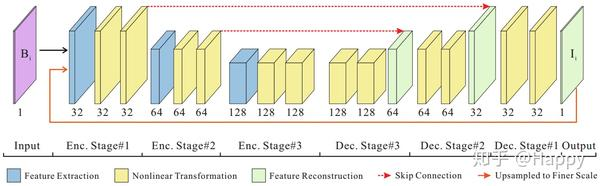### 参数选择性共享

• 什么类型的参数可以进行跨尺度共享；
• 通尺度不同模块的参数是否可以共享。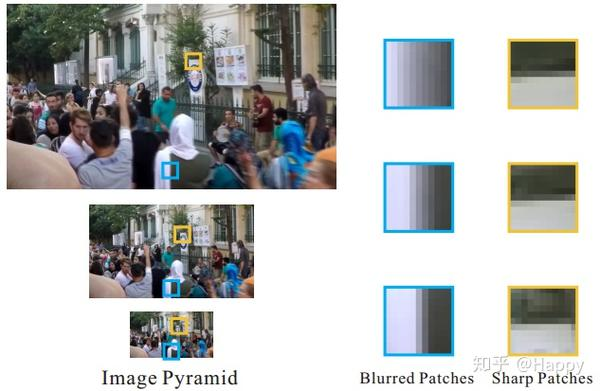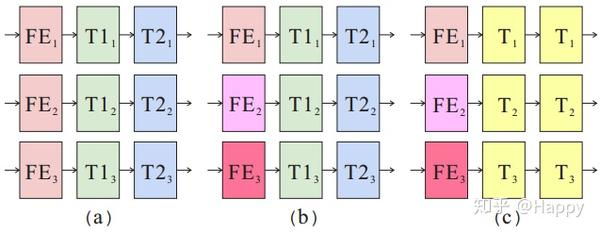### 嵌套跳过连接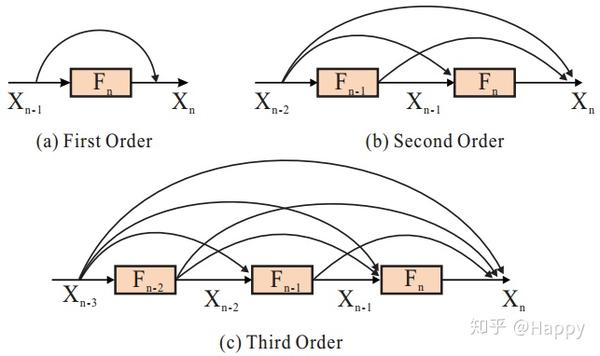• 这里的跳过连接指的是相加而非拼接；
• 直接连接的数量为,而DenseNet中最后一个卷积的连接数则是(L+1).

## Experiments

• 固定相机以确保不存在相机抖动；
• 白天在户外采集视频以确保低噪声；
• 仅收集具有足够细节的场景以避免大面积平滑区域(如天空、背景)。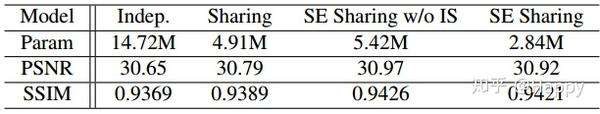• 参数共享优于参数独立(更高的性能、更少的参数量)；
• 选择性参数共享可以进一步提升去模糊性能；
• 尺度内参数共享可以取得与非贡献同比的性能，但参数量仅为其一半。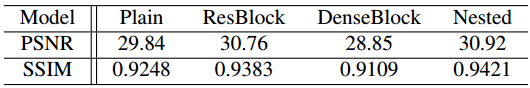• ResBlock由于卷积堆叠；
• Nested与ResBlock均优于DenseBlock；
• Nested取得最佳性能。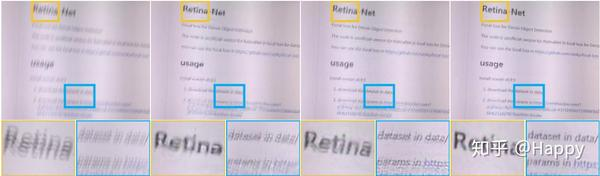## Conclusion

--------------------------------------------------

1. [CVPR2017] Deep Multi-scale Convolutional Neural Network for Dynamic Scene Deblurring.
2. [CVPR2018] Scale-recurrent Network for Deep Image Deblurring.

## 参考代码

# 因作者并未开源，故而不确定NestedBlock具体形式。
# 这里只是参考文中架构图简单的对比了NestedBlock与ResBlock
# 可以与下面的标准残差模块对比分析两者之间的差异。
# 更具体的网路架构这里不再列出
# 看懂了文中架构图，结合NestedBlock以及SRN可以轻松的写出相关架构代码
class Block(nn.Module):
def __init__(self, inc):
super().__init__()
self.conv1 = nn.Conv2d(inc, inc, 3, 1, 1)
self.relu  = nn.ReLU()
self.conv2 = nn.Conv2d(inc, inc, 3, 1, 1)
def forward(self, x):
return self.conv2(self.relu(self.conv1(x)))

class NestedBlock(nn.Module):
def __init__(self, inc):
super().__init__()
self.blk1 = Block(inc)
self.blk2 = Block(inc)
self.blk3 = Block(inc)
self.blk4 = Block(inc)
def forward(self, x):
x1 = self.blk1(x)
x2 = self.blk2(x + x1)
x3 = self.blk3(x + x1 + x2)
x4 = self.blk3(x + x1 + x2 + x3)
return x + x1 + x2 + x3 + x4

class ResBlock(nn.Module):
def __init__(self, inc):
super().__init__()
self.conv1 = nn.Conv2d(inc, inc, 3, 1, 1)
self.relu  = nn.ReLU()
self.conv2 = nn.Conv2d(inc, inc, 3, 1, 1)
def forward(self, x):
res = self.conv2(self.relu(self.conv1(x)))
return res + x

2 阅读 30
##### 0 条评论6

16